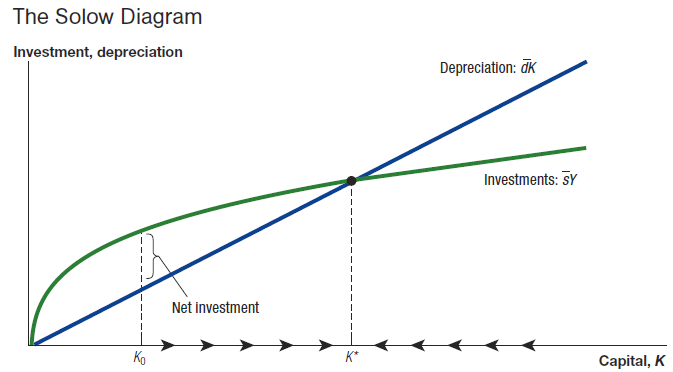# PPE Tutor Oxford – Politics Philosophy Economics Tutor for Oxford

### Oxford University tutors for Microeconomics, Macroeconomics, Econometrics and Finance coursework in Oxford and online. Private one-on-one lessons for economics.Hire our PPE Tutor for Oxford University. Our tutors prepare students for all economics coursework.

#### Exercise on consumption in the Solow growth model

The Solow growth model is in steady-state when investment

$s{Y}_{t}$

is exactly offset by depreciation

$d{K}_{t}$

.

1. Show that, together with the production function
${Y}_{t}=A{K}_{t}^{\frac{1}{3}}{L}_{t}^{\frac{2}{3}}$

this implies that steady-state output per worker is given by: Consumption is subject to the resource constraint

${C}_{t}={Y}_{t}–{I}_{t}$

where by assumption

${I}_{t}=\overline{)s}{Y}_{t}$

. Use these two equations and your earlier result to derive an expression for consumption per worker in steady state.

2. Sketch a plot of how consumption per worker varies in steady state with the investment rate . Explain the intuition for why the plot has the shape that it does.
3. What is the level of s that maximises steady-state consumption per worker? Work graphically with the Solow diagram to identify the optimal investment rate and then use basic calculus on the expression you derived in part iii) to calculate its precise value.

#### Short essay questions

1. In the Solow growth model will an economy that increases its savings rate experience faster growth? How does your answer depend on the properties of the production function?

2. During the 1950s and 1960s, Germany and Japan had much faster rates of economic growth than did the United States. What might account for these differences in growth rates?  Did these differences occur because of a fundamental defect in the U.S. economy?

Macroeconomics Tutor Solow Model Lecture

Other popular courses:

Book a free trial lesson with our PPE Tutor Oxford.

Get Started

## See the #1 economics mentoring platform in action

Request a demo